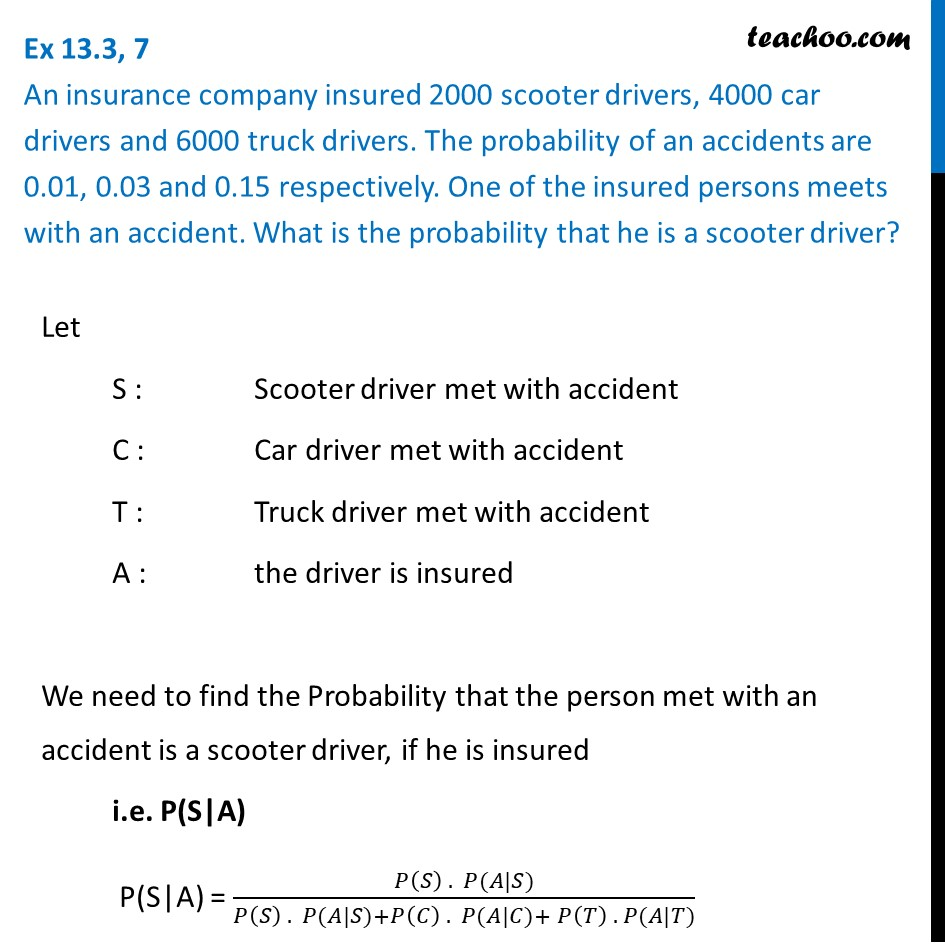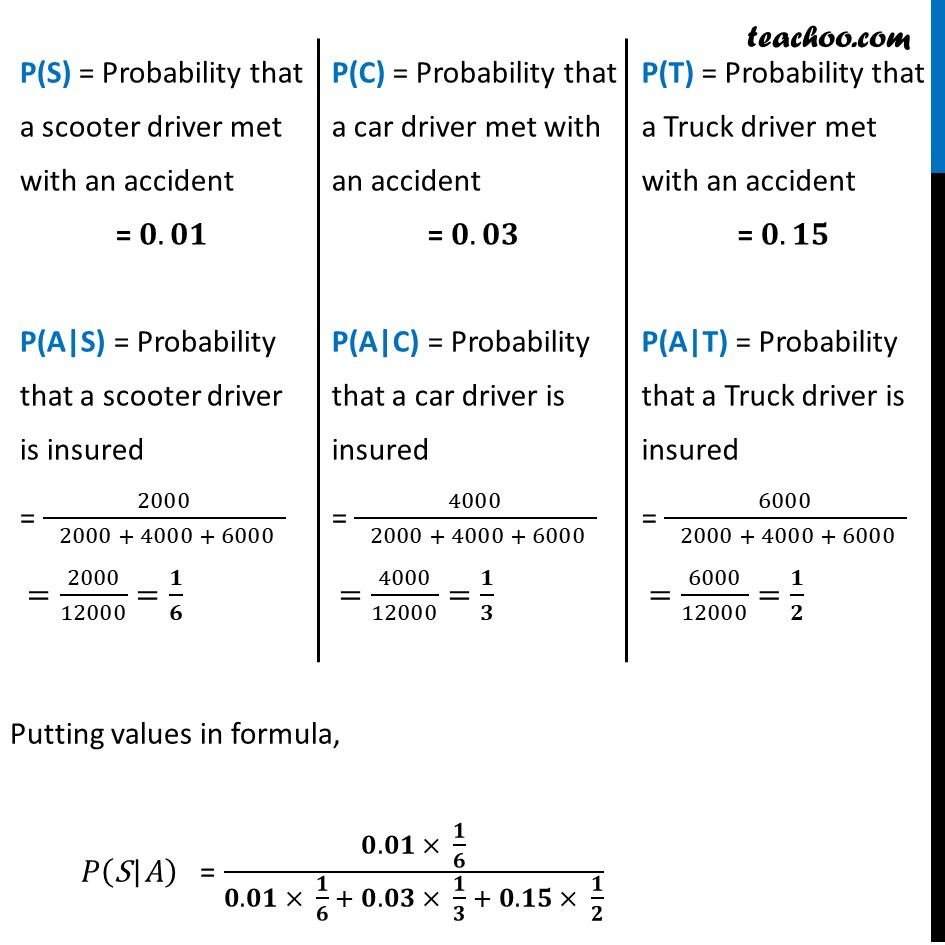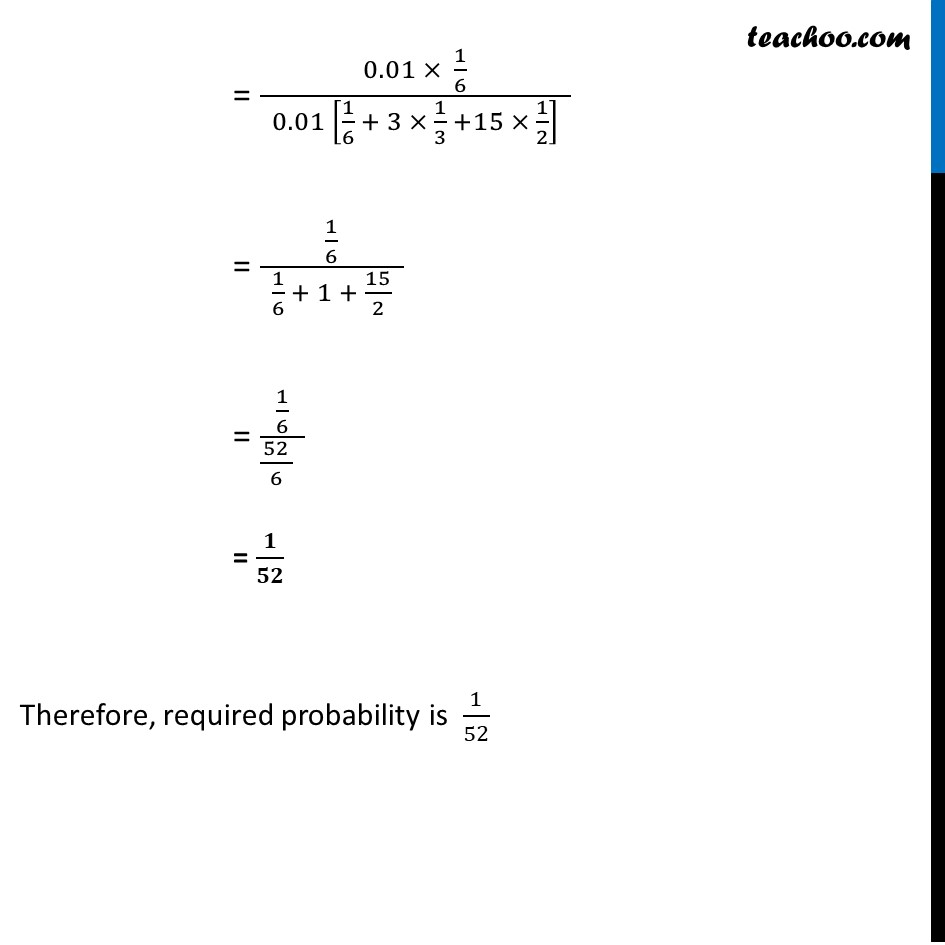Ex 13.3

Chapter 13 Class 12 Probability
Serial order wiseLearn in your speed, with individual attention - Teachoo Maths 1-on-1 Class

### Transcript

Ex 13.3, 7 An insurance company insured 2000 scooter drivers, 4000 car drivers and 6000 truck drivers. The probability of an accidents are 0.01, 0.03 and 0.15 respectively. One of the insured persons meets with an accident. What is the probability that he is a scooter driver?Let S : Scooter driver met with accident C : Car driver met with accident T : Truck driver met with accident A : the driver is insured We need to find the Probability that the person met with an accident is a scooter driver, if he is insured i.e. P(S"|"A) P(S"|"A) = (𝑃(𝑆) . 𝑃(𝐴|𝑆))/(𝑃(𝑆) . 𝑃(𝐴|𝑆)+𝑃(𝐶) . 𝑃(𝐴|𝐶)+𝑃(𝑇) . 𝑃(𝐴|𝑇)) P(S) = Probability that a scooter driver met with an accident = 𝟎.𝟎𝟏 P(A|S) = Probability that a scooter driver is insured = 2000/( 2000 + 4000 + 6000 ) =2000/12000=𝟏/𝟔 P(C) = Probability that a car driver met with an accident = 𝟎.𝟎𝟑 P(A|C) = Probability that a car driver is insured = 4000/( 2000 + 4000 + 6000 ) =4000/12000=𝟏/𝟑 P(T) = Probability that a Truck driver met with an accident = 𝟎.𝟏𝟓 "P(A|T)" = Probability that a Truck driver is insured = 6000/( 2000 + 4000 + 6000 ) =6000/12000=𝟏/𝟐 Putting values in formula, 𝑃("S|" 𝐴) = (𝟎.𝟎𝟏 × 𝟏/𝟔)/(𝟎.𝟎𝟏 × 𝟏/𝟔 + 𝟎.𝟎𝟑 × 𝟏/𝟑 + 𝟎.𝟏𝟓 × 𝟏/𝟐) = (0.01 × 1/6)/( 0.01 [1/6 + 3 × 1/3 +15 × 1/2] ) = (1/6)/( 1/6 + 1 + 15/2 ) = (1/6)/(52/( 6 ) ) = 𝟏/𝟓𝟐 Therefore, required probability is 1/52